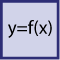# Mathematical FunctionsMath objects with user-defined formulas can execute mathematical functions on data points. A math object uses a number of data points as input variables (v1, v2, …, vn) and calculates a result according to the formula. The result is written as an output to a number of data points. The calculation is executed each time one of the input data points changes its value. A result is calculated only if all inputs have a valid value.